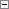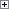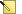In C/AL, some .NET Framework data types, such as strings, DateTime, integers, and decimals, are automatically converted to C/AL types. Because the types are converted, the .NET Framework versions of these types are not supported in C/AL. For example, instead of using a .NET Framework integer data type in your C/AL code, you should use a C/AL integer data type. When the C/AL integer is sent back to a .NET Framework object, such as through a method call, then the C/AL integer is automatically converted to a .NET Framework integer.

Conversion Table for .NET Framework and C/AL Types

The following table lists the automatic data type conversions between.NET Framework and C/AL types.Note
The System.String type and System.DateTime type are converted differently than other types that are listed in the table. For more information, see Conversion of System.String and System.DateTime Types.

.NET Framework Data Type (range) C/AL Data Type (range) Comments

System.Byte

(0...255)

Byte

(0...255)

Single unsigned byte that represents a value from 0...255.

System.SByte

(-128...127)

Integer

(-128...127)

Single signed byte that represents a value from -128...127.

System.Char

(0...65535)

Char

(0...65535)

Unicode character that is represented internally as a 16-bit unsigned integer.

System.Int16

(-32768...32767)

Integer

(±2,147,483,647)

System.Int32

(-2,147,483,648...2,147,483,647)

Integer

(±2,147,483,647)

System.Int64

(-9,223,372,036,854,775,808...9,223,372,036,854,775,807)

BigInteger

(±9,223,372,036,854,775,807)

System.UInt16

(0...65335)

Integer

(±2,147,483,647)

Microsoft Dynamics NAV does not have a corresponding type, but values can be stored in an integer.

System.UInt32

(0...4,250,000,000)

BigInteger

(±9,223,372,036,854,775,807)

Microsoft Dynamics NAV does not have a corresponding type, but values can be stored in a big integer.

System.UInt64

(0...18,446,744,073,709,551,615)

Decimal

(0...18,446,744,073,709,551,615)

Microsoft Dynamics NAV does not have a corresponding type, but values can be stored in a big integer or decimal.

System.Single

(±3.402823e38)

Decimal

(±3.402823e38)

System.Double

(±1.79769313486232e308)

Decimal

(±1.79769313486232e308)

System.Decimal

(±79,228,162,514,264,337,593,543,950,335)

Decimal

(-999,999,999,999,999.99...999,999,999,999,999.99)

An internal range that is not persisted to a field but is the type’s native value range.

System.Int32

(±2,147,483,647)

Option

(±2,147,483,647)

Option values can be freely converted to numeric values. The range is the same as an integer.

System.Enum

(-32768...32767)

Integer

(±2,147,483,647)

An enumeration is a named constant with an underlying type that is any integer type except Char. If no underlying type is explicitly declared, then Int32 is used.

Microsoft Dynamics NAV has no information about the constant’s name, only the value is known.

System.Bool

(TRUE, FALSE)

Boolean

(TRUE, FALSE)

System.String

(0 to 1024 bytes)

Text

(0 to 1024 bytes)

BigText

(up to 2 gigabytes)

Denotes a text string with a maximum length of 1024 characters. Text strings are single-byte only.

For more information, see Conversion of System.String and System.DateTime Types.

System.String

(0 to 1024 bytes)

Code

(0 to 1024 bytes.)

Denotes an alphanumeric string with maximum length of 1024 characters. The value is stored in uppercase.

For more information, see Conversion of System.String and System.DateTime Types.

System.DateTime

3 January year 1 ... 31 December 9999

Date

1 January year 1753 ... 31 December 9999

The common language runtime only supports DateTime. In Microsoft Dynamics NAV, Date must be converted to a DateTime value type when passing as a parameter, such as for CREATEDATETIME(d, 000000T);

For more information, see Conversion of System.String and System.DateTime Types.

System.DateTime

Time

(00:00:00...23:59:59.999)

The common language runtime only supports DateTime. In Microsoft Dynamics NAV, Date must be converted to a DateTime value type when passing it as a parameter, such as for CREATEDATETIME( 0D, t);

The common language runtime DateTime object that will be used for storing a Microsoft Dynamics NAV Time value must handle daylight saving time and time zone. The time value does not change.

For more information, see Conversion of System.String and System.DateTime Types.

System.DateTime

DateTime

(January 1, 1753, 00:00:00.000 to December 31, 9999, 23:59:59.999)

The common language runtime DateTime value type represents dates and times with values ranging from 12:00:00 midnight, January 1, 1753 Common Era (CE) through 11:59:59 P.M., December 31, 9999 CE.

For more information, see Conversion of System.String and System.DateTime Types.

TimeSpan

(Resolution is 100 nanoseconds)

Duration

(Resolution is 1 milliseconds)

A time interval is the duration of time or elapsed time that is measured as a positive or negative number of days, hours, minutes, seconds, and fractions of a second. Duration is internally represented as a 64-bit integer.

System.Guid

(128 bit number)

GUID

(128 bit number)

System.IO.Stream

inStream

Streams require a context specific conversion and specialized stream types such as {Stream,String,Text}{Reader,Writer} classes. BLOB-related streams typically use MemoryStreams.

System.IO.Stream

Outstream

Conversion of System.String and System.DateTime Types

Unlike the types in the previous table, the System.String and System.DateTime types are not converted automatically to C/AL data types when the DotNet variable is instantiated. The System.String and System.DateTime types are only converted when assigned to a compatible C/AL data type. This lets you create an instance of the System.String type or System.DateTime type, and then call the DotNet variable like any other DotNet variable.Note
You cannot use a DotNet variable for the System.String type or System.DateTime type in comparisons with C/AL types because in these cases, there is no implicit type conversion.

Example

The following C/AL code example illustrates how a DotNet variable for the System.String type is converted to a C/AL text data type.

In a Microsoft Dynamics NAV object, such as a codeunit, define the following global variables.

Variable name DataType SubType Comments

alVariable

Text

dotNetVariable

DotNet

System.String

Located in the mscorlib assembly.

In the C/AL code, add the following code.Copy Code
alVariable := ‘sample text’;
dotNetVariable := ‘sample text’;
// Compares the objects using the Equals method on the DotNet object.
if not dotNetVariable.Equals(alVariable) then
error(‘Object should contain same data’);
// Converts the DotNet object to a C/AL text type by calling the ToString method on the object.
// This forces an implicit type conversion to the C/AL text type and the standard C/AL comparison can be used.
if dotNetVariable.ToString() <> alVariable then
error(‘Objects should contain same data, compared with ToString()’);